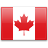S Q T L

S Q T L

S Q T L

S Q T L

S Q T L

S Q T L

S L Q T

S Q L T

S Q L T

S L Q T

S L Q T

S Q L T

S L Q T

S L Q T

S L Q T

S L Q T

S L T Q

S L Q T

S L Q T

S Q L T

S L Q T

S Q T L

L T Q S

L Q T S

T L S Q

L S T Q